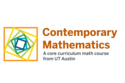Subject:
Mathematics
Material Type:
Activity/Lab, Full Course, Lesson
Level:
Workforce Education (Technical), Academic Lower Division
Provider:
University of Texas at Austin
Tags:
• D2S2
• Game Analysis
• Mindset
• Not Reviewed
• d2s2

# MATH 1332 Introduction to Mathematics (M 302)

This is a terminal course satisfying the University's general education requirement in mathematics. Topics may include: number theory (divisibility, prime numbers, the Fundamental Theorem of Arithmetic, gcd, Euclidean Algorithm, modular arithmetic, special divisibility tests), probability (definition, laws, permutations, and combinations), network theory (Euler circuits, traveling salesman problem, bin packing), game theory.

Unit 1
Unit 1
•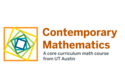•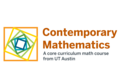Lesson 2

1.2 Puzzles
•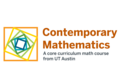•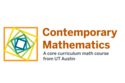•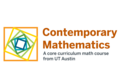Unit 2
Unit 2
•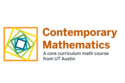•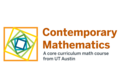•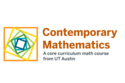•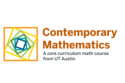•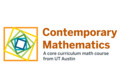•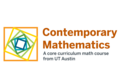Unit 3
Unit 3
•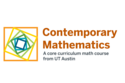•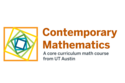•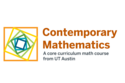•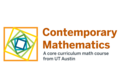•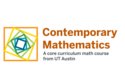•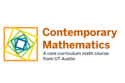•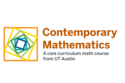Unit 4
Unit 4
•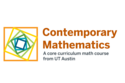Lesson 1

4.1 Topology
•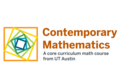•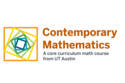•Unit 5
Unit 5
••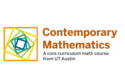•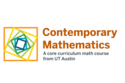•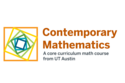•